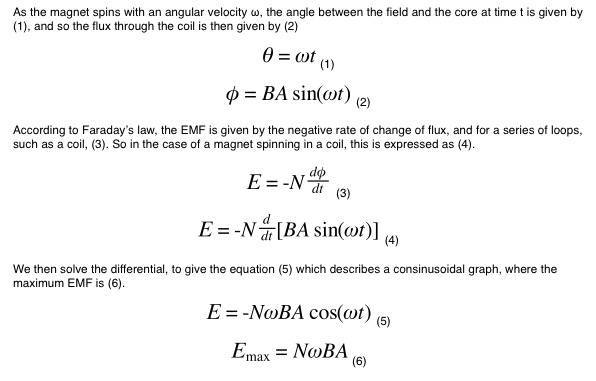# Equation for the EMF generated by a spinning magnet?

• Wilfrid Somogyi

#### Wilfrid Somogyi

I have found an equation which gives the EMF generated in a coil when a magnet is spun near it, the equation is given as:

E = FNAω × 10-8 volts.

Where F is the field of the sample perpendicular to the axis of roation, N is the number of turns, A is the effective area of the coil and ω is the angular velocity of rotation.

Now this looks a lot like Faraday's law, E = N[ΔBA/t].

My question is, how do you get from Faraday's law to the first equation?

Assuming that when they say F is the field, they mean the strength of the field, I don't see how you can get the units to match up. Unless I'm mistaken and F is more than just the strength of the field and it is perhaps a vector quantity, but then what vector quantity is it and how is it desribed?

Update: I think I've sussed it, though if anyone can verify my mathematics that would be great.